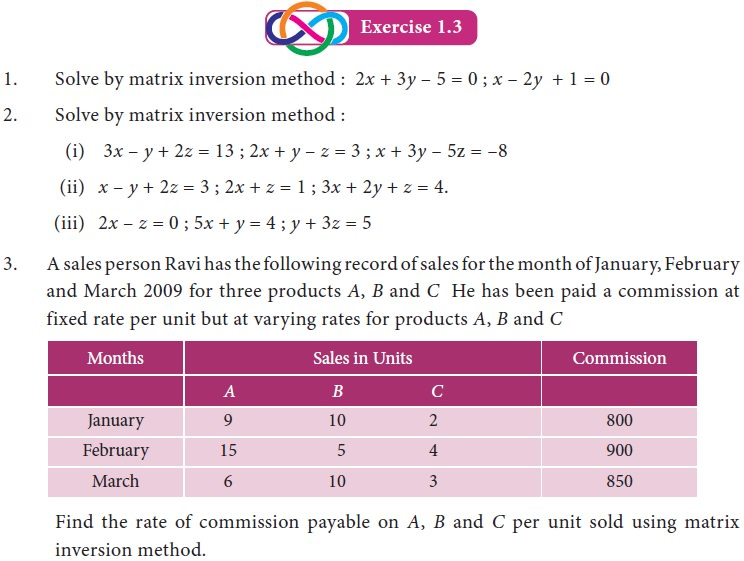Home | | Business Maths 11th std | Inverse of a matrix

# Inverse of a matrix

Business Mathematics and Statistics(EMS) : Matrices and Determinants

Inverse of a matrix

## 1. Singular matrix

A Square matrix A is said to be singular, if  A = 0

## 2. Non – singular matrix:

A square matrix A is said to be non – singular, if  A Î 0## 3. Adjoint of a Matrix

The adjoint of a square matrix A is defined as the transpose of a cofactor matrix. Adjoint of the matrix A is denoted by adj A## 4. Inverse of a matrix

Let A be any non-singular matrix of order n. If there exists a square matrix B of order n such that AB = BA = I then, B is called the inverse of A and is denoted by A-1## 5. Solution of a system of linear equations

Consider a system of n linear non – homogeneous equations with n unknowns x1 , x2, ......xn  asExample 1.22

The cost of 4 kg onion, 3 kg wheat and 2 kg rice is `320. The cost of 2kg onion, 4 kg wheat and 6 kg rice is `560. The cost of 6 kg onion, 2 kg wheat and 3 kg rice is `380. Find the cost of each item per kg by matrix inversion method.Tags : Definition, Formula, Solved Example Problems, Exercise | Mathematics , 11th Business Mathematics and Statistics(EMS) : Chapter 1 : Matrices and Determinants
Study Material, Lecturing Notes, Assignment, Reference, Wiki description explanation, brief detail
11th Business Mathematics and Statistics(EMS) : Chapter 1 : Matrices and Determinants : Inverse of a matrix | Definition, Formula, Solved Example Problems, Exercise | Mathematics